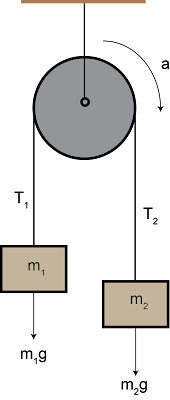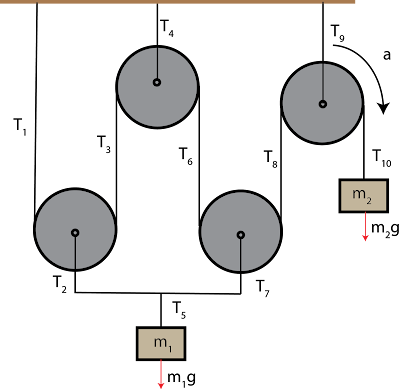## The SliderPulley problems (also called Atwood machine) are the favorite problems to the professors and students seem to really struggle with it. There are several ways to solve it and some of them are too complicated to understand. But  here I will try to explain a general and easier way to approach the problem.

#### Case I: Massless pulley

Simple Pulley (Atwood machine)
Suppose a string is placed over a massless and frictionless pulley. A block with mass $m_1$  is suspended at one end while another block with mass of $m_2$ is suspended from the other end. Like the figure below-Say $m_1<m_2$. Then it is obvious that the pulley will move in a clockwise direction (look at the figure). Now how can you calculate the acceleration of the system?
Solution:
From Newton's law we can write-
$$F_{net}=Ma$$
Right? And then we can rewrite it as-
$$a=\dfrac{F_{net}}{M}$$
Awesome, it wasn't that hard. Only things we need to figure our is the $M$ and  $F_{net}$. Since the pulley is massless (according to the question), we only need to consider the mass of the two blocks. i.e.
$$M=m_1+m_2$$
Now consider the mass $m_1$, the only force acting on it is the gravitational force.
$$F_1=m_1g$$
What about the mass $m_2$ ?  It's
$$F_2=m_2g$$
Now since  $m_1<m_2$, $F_2$ will go downwards and $F_1$ will move upward (see the figure). If we consider the downward direction as positive then $F_2$ would be positive, $F_1$ would be negative, make sense? Our $F_{net}$  will be
$$F_{net}=m_2g-m_1g$$.
Great we have found both $M$ and  $F_{net}$, you just need to plug these to the equation
\begin{align*} a &=\dfrac{F_{net}}{M}\\ \Rightarrow a &=\dfrac{m_2g-m_1g}{m_1+m_2} \end{align*}
Now what if the professor asks you to calculate the Tensions on the strings?
You can start from the scratch and derive the whole formula but during the exam it would take a lot of time. The easiest way is to remember these two equations-
\begin{align*}
T-mg &= ma \qquad\text{ if $m$ goes up}\\
mg-T &= ma \qquad\text{ if $m$ goes down}
\end{align*}

In our case $m_1$ is going up Going up, so the tension $T_1$ is-
$$T_1-m_1g= m_1a$$

And $m_2$ is going down, so the tension $T_2$ is-
$$m_2g-T_2=m_2a$$
Solve for $T_1$ and $T_2$, the total Tension would be $$T=T_1+T_2$$

#### Case- II: System with Two Pulleys (Atwood machine)

Now, consider a little bit harder problem, you have two pulleys and they are in equilibrium. What is the tension force at each section of the rope?
Solution:From the figure it is clear that
$$T_2=m_1g$$
Now look closely both $T_1$ and $T_3$ are sharing the weight $m_1g$, that means $m_1g$ is evenly distributed between $T_1$ and $T_3$ $$T_1=\dfrac{m_1g}{2}=T_3$$ Since the system is in equilibrium, $T_5$ should apply the same amount of force as $T_3$. i.e $$T_5=T_3=\dfrac{m_1g}{2}$$
What about $T_4$? Tension $T_4$ actually holding the both $T_3$ and $T_5$ in equilibrium. So, it will be-
\begin{align*} T_4 &=T_3+T_5\\
&=\dfrac{m_1g}{2}+\dfrac{m_1g}{2}\\
&=m_1g\\
\end{align*}
And Boom! you have all the tension forces.

#### Case III: System with Two pulleys with weight at the End

Okay, let's add another weight to the system and consider $m_1<m_2$. Now the system has acceleration and when the block $m_2$ goes down with acceleration $a_2$, block $m_1$ moves up with the acceleration $a_1$.  How do you calculate these accelerations? And also does the tension change in this situation?Solution:
Like the first example we can use the Newton's law but we have two pulleys now. So let's apply $F=ma$ for the block $m_2$, we have two forces acting on it, force due to gravity which is acting downwards (which means ) and the tension force. So-
\begin{align*}
F_{net}&=m_{2}a_{2}\\
\Rightarrow T_{5}-m_2g&=m_{2}a_{2}---(A)
\end{align*}
Now consider the block $m_1$. There are three forces acting on it: $T_2=m_1g$, $T_1$ and $T_2$. So-
\begin{align*}
F_{net}&=m_{1}a_{1}\\
\Rightarrow T_{1}+T_{2}+T_{3}&=m_{1}a_{1}\\
\Rightarrow T_{1}+m_1g+T_{3}&=m_{1}a_{1} \\
\end{align*}
But wait, $T_3=\dfrac{(m_1+m_2)}{2}g$. And we know (from previous example) $T_3=T_1$
\begin{align*}
T_{1}+T_{2}+T_{3}&=m_{1}a_{1}\\
\Rightarrow \dfrac{m_1+m_2}{2}g+m_1g+\dfrac{m_1+m_2}{2}g&=m_{1}a_{1}\\
\Rightarrow 2m_{1}g+m_2g&=m_{1}a_{1}\\
\Rightarrow a_{1}&=\dfrac{2m_{1}g+m_2g}{2}

\end{align*}
Now on equation (A) using $T_3=T_5$
\begin{align*}

T_{5}-m_2g&=m_{2}a_{2}\\
\Rightarrow \dfrac{(m_1+m_2)}{2}g-m_2g&=m_{2}a_{2}\\
\Rightarrow \dfrac{(m_1m_2)}{2}g&=m_{2}a_{2}\\
\Rightarrow a_{2}&= \dfrac{(m_1-m_2)}{2m_2}g\\
\end{align*}

#### Case- IV: Pulley with weight:

Till now we have only consider that the pulley itself is massless. Just for fun, let's say we have the same configuration as example 1 but this time pulley has some mass $m_p$ and the radius is $r$. How can you calculate the acceleration? Before proceeding note that, to solve this type of problem (pulley with mass) you need to know about 'Inertia' and 'Rotational motion'. If your professor didn't cover that, you won't see this problem in your midterm. Anyway, now the figure looks something like this:We know for a rotational object acceleration is-
$$a=\alpha r$$
And as the previous examples using Newton's law for $m_1$ we can write-
$$T_1-m_1g=m_1 a$$
And for $m_2$ we have
$$m_2g-T_2=m_2 a$$
Since this time our pulley has the mass, we need to consider the net force acting on the pulley too. Using the laws of rotational motion, we can write-
$$T_2r-T_1r=I\alpha=\dfrac{I}{r}a$$
Adding all the above equation we can solve for $a$:
$$a=\dfrac{(m_2-m_1)g}{m_1+m_2+I/r^2}$$
And that's your equation for acceleration for the massive pulley system.

Does the above example make sense? Let's check. It's your turn now.  Say you have four pulleys and as before $m_1<m_2$.Now calculate the acceleration and the tension force for this system for these cases
1) What if the pulleys has no mass?
2) What happens if you consider that the pulleys have some masses?

Let me know in the comments.

How to solve the pulley problems (hanging from the ceiling)Reviewed by Dayeen on 1:22:00 AM Rating: 5Hello, my name is Jack Sparrow. I'm a 50 year old self-employed Pirate from the Caribbean.